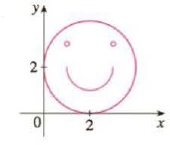Chapter 10.1, Problem 35E

Chapter
Section
Textbook Problem

# Use a graphing calculator or computer to reproduce the picture.35.To determine

To plot: The reproduce the graph.

Explanation

The big circle has the center (2,2) with the radius of 2.

The parametric equation for the variable x is as follows:

x=2+2cost (1)

The parametric equation for the variable y is as follows:

y=2+2sint (2)

The value of t is increased from 0 to 2π with a step value of π2 and substituted in the Equations (1) and (2) to obtain the value of x and y respectively.

Substitute π for t in the Equation (1).

x=2+2cost=2+2cos(π)x=0

Substitute π for t in the Equation (2).

y=2+2sint=2+2sin(π)y=2

The values of x and y for each step value of t is tabulated in the below table.

 t 0 π 3π2 2π x 4 0 2 4 y 2 2 0 2

The left side small circle has the center (1,3) with the radius of 0.1 .

The parametric equation for the variable x.

x=1+0.1cost (3)

The parametric equation for the variable x.

y=3+0.1sint (4)

The value of t is increased from 0 to 2π with a step value of π2 and substituted in the Equations (3) and (4) to obtain the value of x and y respectively.

Substitute π for t in the equation (3),

x=1+0.1cost=1+0.1cos(π)x=0.9

Substitute π for t in the equation (4),

y=3+0.1sint=3+0.1sin(π)y=3

The values of x and y for each step value of t is tabulated in the below table.

 t 0 π 3π2 2π x 1.1 0.9 1 1.1 y 3 3 2.9 3

The right side small circle has the center (3,3) with the radius of (0.1) .

The parametric equation for the variable x.

x=3+0.1cost (5)

The parametric equation for the variable x

### Still sussing out bartleby?

Check out a sample textbook solution.

See a sample solution

#### The Solution to Your Study Problems

Bartleby provides explanations to thousands of textbook problems written by our experts, many with advanced degrees!

Get Started